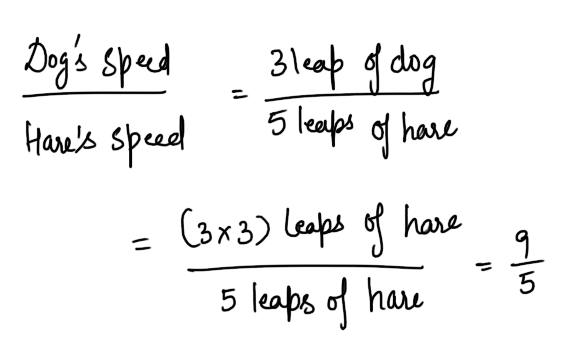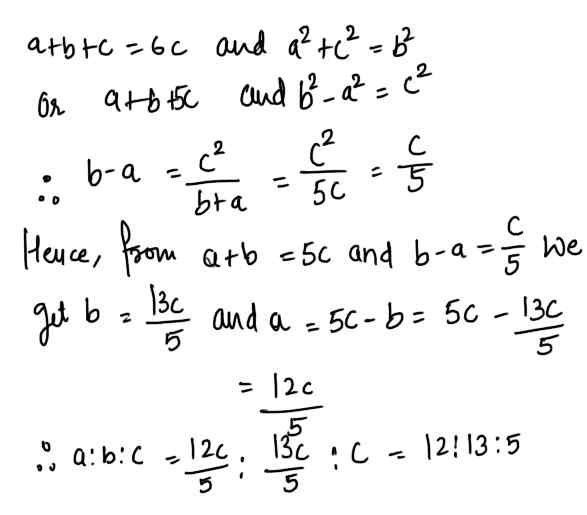# Mixed Aptitude Quiz For RBI Grade B : Part 3#### Ques 1.

If ( k + 1) represents an odd integer, which of the following must also be an odd integer ?
(a) 2 ( k + 1)
(b) k (k + 1)
(c) ( k+1) ( k + 2)
(d) ( k + 1)( k - 1)
Ans 1.  K +1 -> odd; K -> even
Now,2 (K + 1)  - > even; K ( k + 1) ->   even;
( K+ 1) ( K + 2)  --> even; ( K+1) ( k -1)  -> odd

#### Ques 2.

A boy was asked to write the value of 25 x 92. He wrote it as 2592. The difference between the obtained value and actual value is
(a) 21 x 92
(b) 22 x 93
(c) 23 x 94
(d) Zero

Ans 2. 2592 = 25 x 34
. . 2592 - 25 x 92 = 25 x 34 - 25 x 34 = 0

#### Ques 3.

Subtracting 40% of a number from the number, we get the result as 30. The number is
(a) 28
(b) 60
(c) 52
(d) 70
Ans 3.  x - 40/100x = 30  or  60/100x = 30
=>  x = 50

#### Ques 4.

3/126 correct to 5 places of decimal is
(a) 5.01329
(b) 5.51565
(c) 5.11329
(d) 5.21319
Ans 4. The choice is obvious.

#### Ques 5.

A number exceeds 50 by 20% .The number is
(A) 90
(b) 20
(c) 70
(d) 60
Ans 5. x -50/50 x 100 = 20
=> x = 60

#### Ques 6.

What number should be added to each of the numbers 8, 21, 13 and 31 as that the resulting number in this order forms a proportion?
(a) 2
(b) 3
(c) 5
(d) 7
Ans 6#### Ques 7.

The number which when multiplied by itself exceeds half a century by a dozen plus two is
(a) 8
(b) 16
(c) 4
(d) 10
Ans 7.#### Ques 8.

A dog takes 3 leaps for every 5 leaps of a hare. If one leap of the dog is equal to 3 leap of the hare, the ratio of the speed of the dog to that of the hare is
(a) 8 : 5
(b) 9 : 5
(c) 8 : 7
(d) 9 : 7
Ans 8.#### Ques 9.

The perimeter of a right- angled triangle is six times the length of its shortest side. The ratio of the sides are
(a) 5 : 4 : 3
(b) 10 : 8 : 6
(c) 11 : 10 : 4
(d) 13 : 12 : 5
Ans 9.#### Ques 10.

The mean of 13, 23, 33, 43, 53, 63, and 73, is
(a) 412
(b) 784
(c) 112
(d) 222
Ans 10.